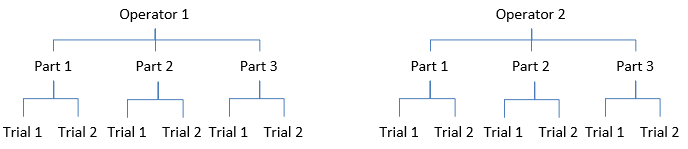## What is a Gage R&R Study?

This is the first of a four-part blog on gage R&R. It answers this basic question:

What is a Gage R&R study?

If you google this question, you will get several answers including this one from the www.isixsigma.com dictionary:

“Gage R&R, which stands for gage repeatability and reproducibility, is a statistical tool that measures the amount of variation in the measurement system arising from the measurement device and the people taking the measurement.”

This statement is true – the methodology will give you the value for variation due to the measurement system and the people taking the measurement. But you need to compare this value to something to answer the question of “how good” your measurement system is.

For any process, the total process variance is the sum of the parts variance and the measurement system variance.

σx2= σp2e2

where σx2= total variance of the product measurements, σp2 = the variance of the product, and σe2 = the variance of the measurement system. We start by examining how a Gage R&R study determines the variance of a measurement system.

Suppose your Gage R&R has two operators who will measure each of three parts two times. A diagram of this Gage R&R is shown below.Consider Operator 1 who measures part 1 two times. Maybe he is using calipers to measure the length of a part. What are the sources of variation in those two results? It is the same part and the same operator. The only source of variation is the measurement system itself (the calipers). This is often called the test-retest error. In this setup, you have six estimates of this test-retest error. This is the repeatability of the measurement system. It measures how repeatable the results are when a part is remeasured multiple times.

Now average the results for Operator 1 and Operator 2. What are the sources of variation present in the operator averages? The test-retest method is present since there are two trials for each part. The other source of variation is the operators themselves. You then can determine the reproducibility of the measurement method from the difference in operator averages (and accounting for the presence of the test-retest error).

Combining the repeatability and reproducibility results gives you the variance of the measurement system (σe2 ). This is the key measurement for determining “how good” the measurement system is.

• Is the measurement system capable of telling the difference between the parts/samples?
• Is the measurement system capable of telling if a part/sample is within specification?

Our next blog will show how these questions are answered based on the Gage R&R results.

### Filtered HTML

• Allowed HTML tags: <a> <em> <strong> <cite> <code> <ul> <ol> <li> <dl> <dt> <dd> <h1> <h2> <h3> <h4> <h5> <h6> <img> <hr> <div> <span> <strike> <b> <i> <u> <table> <tbody> <tr> <td> <th>
• Lines and paragraphs break automatically.

### Plain text

• No HTML tags allowed.
• Lines and paragraphs break automatically.
This question is for testing whether you are a human visitor and to prevent automated spam submissions.